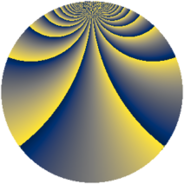# Properties

 Label 900.3.bhLevel $900$ Weight $3$ Character orbit 900.bh Rep. character $\chi_{900}(287,\cdot)$ Character field $\Q(\zeta_{20})$ Dimension $960$ Sturm bound $540$

# Related objects

## Defining parameters

 Level: $$N$$ $$=$$ $$900 = 2^{2} \cdot 3^{2} \cdot 5^{2}$$ Weight: $$k$$ $$=$$ $$3$$ Character orbit: $$[\chi]$$ $$=$$ 900.bh (of order $$20$$ and degree $$8$$) Character conductor: $$\operatorname{cond}(\chi)$$ $$=$$ $$300$$ Character field: $$\Q(\zeta_{20})$$ Sturm bound: $$540$$

## Dimensions

The following table gives the dimensions of various subspaces of $$M_{3}(900, [\chi])$$.

Total New Old
Modular forms 2944 960 1984
Cusp forms 2816 960 1856
Eisenstein series 128 0 128

## Trace form

 $$960q + O(q^{10})$$ $$960q - 16q^{10} - 112q^{22} - 32q^{28} - 16q^{37} + 216q^{40} + 488q^{52} + 400q^{58} - 464q^{70} + 224q^{73} + 400q^{82} - 448q^{85} + 2096q^{88} + 400q^{94} - 640q^{97} + O(q^{100})$$

## Decomposition of $$S_{3}^{\mathrm{new}}(900, [\chi])$$ into newform subspaces

The newforms in this space have not yet been added to the LMFDB.

## Decomposition of $$S_{3}^{\mathrm{old}}(900, [\chi])$$ into lower level spaces

$$S_{3}^{\mathrm{old}}(900, [\chi]) \cong$$ $$S_{3}^{\mathrm{new}}(300, [\chi])$$$$^{\oplus 2}$$